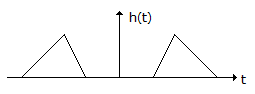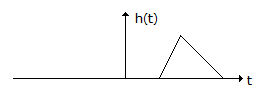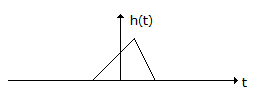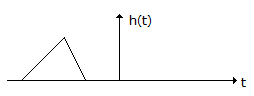# Electronics and Communication Engineering - Signals and Systems

Exercise : Signals and Systems - Section 7
1.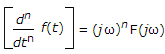True
False
Explanation:
No answer description is available. Let's discuss.

2.
If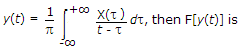-j sgn (f) x X(f)
j2pf x ejptf X(f)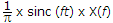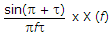Explanation:
No answer description is available. Let's discuss.

3.
Which of the following is/are not a property/properties power spectral density function Sx(ω)?
Sx(ω) is real function of ω
Sx(ω) is a even function of ω
Sx(ω) is non-positive function of ωSx(ω) ≤ 0 for all ω
All of the above
Explanation:
No answer description is available. Let's discuss.

4.
A casual LTI system is described by the difference equation
2y[n] = ay[n - 2] - 2x[n] + βx[n -1]
The system is stable only if
|a| = 2 |β| < 2
|a| > 2 |β| > 2
|a| < 2 any value of β
|β| < 2, any value of a